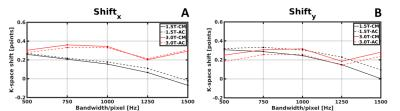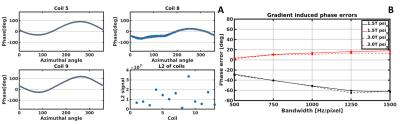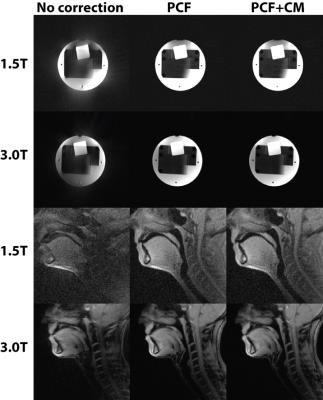### 4012

The efficacy of existing k-space correction methods for 2D golden angle radial sampling on clinical 1.5T and 3T systems
Tom Bruijnen1, Bjorn Stemkens1, Jan J. W. Lagendijk1, Cornelis A. T. van den Berg1, and Rob H. N. Tijssen1

1Center for image sciences, University Medical Center Utrecht, Utrecht, Netherlands

### Synopsis

Golden angle radial acquisitions are sensitive to gradient imperfections leading to reduced image quality. Multiple methods are known to compensate for the zeroth and first order gradient waveform imperfections. Here we quantify the effect of these different methods on Philips 1.5T and 3T wide bore Ingenia scanners, and assess four direct correction methods on efficacy. We show that on these systems a gradient delay correction has minimal impact on the image quality, while phase correction provides a considerable improvement.

### Introduction

Golden angle radial encoding is sensitive to gradient-imperfections, such as finite accuracy in gradient timing and eddy currents, leading to reduced image quality1,2. This has two major consequences: 1) The first order component of these gradient-imperfections results in a shift in k-space along the direction of the readout. This is known to induce considerable artefacts on various fields strengths3,4. 2) The zeroth order component leads to undesirable phase accumulation in k-space and was reported to significantly affect image quality on high field strengths5. The significance of the different sources of imperfections is system dependent and in some cases only investigated on high field preclinical scanners. Here we characterize the size of these effects on Philips 1.5T and 3T wide bore Ingenia scanners for various receiver bandwidths (BW), and assess four direct correction methods on efficacy3–5. We show that on these systems a gradient-delay correction (1st-order) has minimal impact on the image quality, while phase correction (0th-order) causes a considerable improvement.

### Methods:

The 1st-order component of the gradient-imperfection represents effective gradient timing delays, which can be estimated by investigating anti-parallel spokes from a pre-scan3 or directly from the imaging data4. The first method, termed the Calibration Method (CM), acquires a small number of perpendicular and opposing spokes and performs a cross correlation analysis on the phase of the projections to estimate the shift $( \triangle k )$ in the x-direction $(shift_x)$ and y-direction $(shift_y)$. The second method, termed the Autocalibrated Method (AC), computes the shifts by analyzing the phase of all the projections from the imaging and performing a least-squares minimization to the model $\triangle k(\theta) = shift_x \cos (\theta) + shift_y \sin (\theta)$. Here $\theta$ is the azimuthal angle of the readout. Subsequently, each line is corrected with an individual phase shift derived from the model.

The gradient-induced phase errors can be estimated using the azimuthal dependency of the accumulated phase $\varphi (\theta)$(Figure2)5. The phase accumulation of the center point of each radial readout is fitted to the model $\varphi (\theta)=\psi_x \cos (\theta) + \psi_y \sin (\theta) + \varphi_o$ to extract the compensation coefficients $\psi_x$ and $\psi_y$. The correction can be applied by subtracting the accumulated phase from the readout, i.e. multiplying with $\exp( -i(\psi_x \cos (\theta) + \psi_y \sin (\theta)))$, called the Phase Correction Fit (PCF) method. Alternatively, the phase of the central k-point can be subtracted from the readout, i.e. without any underlying model, termed the Phase Correction Zero (PCZ) method.

### Imaging

A 2D SPGR golden angle radial sequence was implemented on a 1.5T and 3T MR scanner (Ingenia, Philips, Best, Netherlands). The sequence was evaluated for a range of BWs (500-1500 Hz/pixel, i.e. Gmax=8-23 mT/m) in a phantom (Matrix=256x256; Pixel size=1.5x1.5mm2; slice thickness=10mm; flip angle 10°; TR/TE=4.1/1.78 ms; axial orientation). Further, the effect of the corrections was demonstrated in two volunteers with BW=1300 Hz/pixel (Matrix=128x128; Pixel size=2x2mm2; slice thickness=10mm; flip angle 10°; TR/TE=4.1/1.78 ms; sagittal orientation). Estimated gradient delays and phase errors were averaged over the receiver channels by L2-weighting. Reconstruction was performed using a non-uniform fast Fourier Transform6 and a sum-of-squares channel combination. The quality of the images was investigated by computing the structural similarity (SSIM)7 and summing the signal intensity in the background (BSI) and comparing it to the gold standard (Cartesian scan, average of two scans with opposing readout direction, equivalent sequence parameters as the radial scan).

### Results & discussion

The estimated gradient delays were small (<0.5 sample point) on both 1.5T and 3T phantom experiments for both gradients and across the complete range of investigated receiver bandwidths (Figure1). The gradient delays behaved according to the proposed sinusoidal model. Results obtained by the CM and AC methods were very similar. The gradient-induced phase errors varied according to the azimuthal angle of the readout and caused phase differences up to 60 degrees (Figure2). A clear linear trend can be observed between the quantified gradient-errors ($\psi_x$ and $\psi_y$) and the receiver bandwidth, becoming larger with increasing bandwidth. Figure3 shows the differences in image quality with the proposed corrections in the phantom and volunteer scans. SSIM and BSI (Table1) showed that phase correction considerably improved both metrics, while gradient delay corrections only had a minor effect.

### Conclusion

Gradient delays were found to be small across the investigated receiver bandwidths on both 1.5T and 3T. Gradient delay corrections therefore not yielded no noticeable improvements in image quality. Gradient-induced phase errors were significant, even on these lower field strengths, contrary to previous published work4,5. Both phase correction methods yielded similar results and can considerably improve image quality.

### Acknowledgements

No acknowledgement found.

### References

1. S. Winkelmann, T. Schaeffter, T. Koehler, H. Eggers, and O. Doessel, “An optimal radial profile order based on the golden ratio for time-resolved MRI,” IEEE Trans. Med. Imaging, vol. 26, no. 1, pp. 68–76, 2007.

2. K. T. Block, M. Uecker, and J. Frahm, “Undersampled radial MRI with multiple coils. Iterative image reconstruction using a total variation constraint,” Magn. Reson. Med., vol. 57, no. 6, pp. 1086–1098, 2007.

3. K. Block and M. Uecker, “Simple method for adaptive gradient-delay compensation in radial MRI,” Proc. Int. Soc. Magn. Reson. Imaging, vol. 19, p. 2816, 2011.

4. G. Buonincontri, C. Methner, T. Krieg, T. A. Carpenter, and S. J. Sawiak, “Trajectory correction for free-breathing radial cine MRI,” Magn. Reson. Imaging, vol. 32, no. 7, pp. 961–964, 2014.

5. A. Moussavi, M. Untenberger, M. Uecker, and J. Frahm, “Correction of gradient-induced phase errors in radial MRI,” Magn. Reson. Med., vol. 71, no. 1, pp. 308–312, 2014.

6. S. J. Vannesjo, M. Haeberlin, L. Kasper, M. Pavan, B. J. Wilm, C. Barmet, and K. P. Pruessmann, “Gradient system characterization by impulse response measurements with a dynamic field camera,” Magn. Reson. Med., vol. 69, no. 2, pp. 583–593, 2013.

7. L. Greengard and J.-Y. Lee, “Accelerating the Nonuniform Fast Fourier Transform,” SIAM Rev., vol. 46, no. 3, pp. 443–454, 2004.  Z. Wang, A. C. Bovik, H. R. Sheikh, and E. P. Simoncelli, “Image quality assessment: From error visibility to structural similarity,” IEEE Trans. Image Process., vol. 13, no. 4, pp. 600–612, 2004.

8. Z. Wang, A. C. Bovik, H. R. Sheikh, and E. P. Simoncelli, “Image quality assessment: From error visibility to structural similarity,” IEEE Trans. Image Process., vol. 13, no. 4, pp. 600-612, 2004.

### FiguresFigure 1: Estimated gradient delays for both in-plane gradients (A+B) for a range of receiver bandwidths. Gradient delays were estimated with the CM and the AC method.Figure 2: (A) Azimuthal angle dependency of the phase error across multiple channels. Note that the model (orange line) fits the data (blue dots) well for channels 5 and 9, but it performs inferior for channel 8. This can be attributed to the low sensitivity of the channel, as seen in the L2-plot. (B) $\psi_x$ and $\psi_y$ scale with increasing bandwidth to a maximum of +20 and -60 degree.Figure 3: Effect of phase corrections and gradient delay corrections on the image quality. First column is without any data correction. Second column is with one of the proposed phase correction methods. Third column is both the phase correction and gradient delay correction. Note that the phantom images are windowed to enhance the appearance of the artefacts, volunteer images are not.Table 1: Quantification of image quality for the various correction methods. Higher SSIM and lower BSI values indicates better quality.

Proc. Intl. Soc. Mag. Reson. Med. 25 (2017)
4012